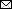### Analysis of Anthrax Immune Globulin Intravenous with Antimicrobial Treatment in Injection Drug Users, Scotland, 2009–2010

Xizhong Cui1, Leisha D. Nolen1, Junfeng Sun, Malcolm Booth, Lindsay Donaldson, Conrad P. Quinn, Anne E. Boyer, Katherine A. Hendricks, Sean Shadomy, Pieter Bothma, Owen Judd, Paul McConnell, William A. Bower, and Peter Q. EichackerAuthor affiliations: National Institutes of Health, Bethesda, Maryland, USA (X. Cui, J. Sun, P.Q. Eichacker); Centers for Disease Control and Prevention, Atlanta, Georgia, USA (L.D. Nolen, C.P. Quinn, A.E. Boyer, K. Hendricks, S. Shadomy, W.A. Bower); Glasgow Royal Infirmary, Glasgow, UK (M. Booth, L. Donaldson); James Paget University Hospital, Norfolk, UK (P. Bothma); Royal Derby Hospital, Derby, UK (O. Judd); Crosshouse Hospital, Kilmarnock, UK (P. McConnell)

Main Article

#### Table 4

Initial laboratory findings of AIG-IV recipients and nonrecipients, Scotland, UK, 2009–2010*

Laboratory test [reference value] Non-AIG-IV AIG-IV p value
Complete blood counts and differentials
Leukocytes, × 109 cells/L, median (IQR) [4–11 × 109/L] 10.9 (8.6–14.1), n = 27 18.9 (9.5–23.2), n = 15 0.02
Neutrophils, × 109 cells/L, median (IQR) [2–7 × 109/L] 7.6 (5.2–10.0), n = 26 15.4 (7.4–19.5), n = 15 0.008
Lymphocytes, × 109 cells/L, median (IQR) [1–3 × 109/L] 1.8 (1.3–2.5), n = 25 2.0 (1.3–2.7), n = 15 0.78
Hemoglobin, g/dL, mean ± SE [12–18 g/dL] 14.0 ± 0.8, n = 27 14.8 ± 1.5, n = 15 0.61
Hematocrit, %, mean ± SE [35%–50%] 41 ± 2, n = 23 42 ± 4, n = 13 0.86
Platelets, × 109/L, mean ± SE [150–450 × 109/L]
214 ± 19, n = 24
181 ± 24, n = 15
0.29
Coagulation parameters and C-reactive protein
Prothrombin time(s), median (IQR) [11–13.5 s] 11.0 (10.0–14.0), n = 15 12.8 (12.0–15.0), n = 14 0.94
Partial thromboplastin time(s), median (IQR) [25–35 s] 26.7 (24.0–36.0), n = 10 33.8 (30.0–39.0), n = 14 0.63
International normalized ratio, median (IQR) [0.8–1.1] 1.1 (1.0–1.3), n = 11 1.1 (1.0–1.3), n = 11 0.36
C-reactive protein, nmol/L, median (IQR) [<95 nmol/L]
21 (8–49), n = 25
32 (17–52), n = 14
0.24
Serum electrolytes and glucose
Sodium, mmol/L, median (IQR) [135–145 mmol/L] 137 (132–139), n = 27 135 (131–136), n = 15 0.11
Chloride mmol/L, median (IQR) [96–108 mmol/L] 100 (96–101) , n = 13 102 (101–103), n = 14 0.76
Potassium, mmol/L, mean ± SE [3.5–5.3 mmol/L] 4.26 ± 0.13, n = 28 4.35 ± 0.22, n = 13 0.69
Calcium, mmol/L, median (IQR) [2.25–2.5 mmol/L] 2.3 (2.0–2.3), n = 28 2.1 (2.0–2.3), n = 12 0.97
HCO3- , mmol/L, mean ± SE [22–28 mmol/L] 24.4 ± 1.2, n = 11 20.7 ± 0.9, n = 11 0.02
Glucose, mmol/L, median (IQR) [3.6–6.0 mmol/L]
6.5 (5.6–8.1), n = 16
7.8 (5.3–8.9), n = 10
0.69
Renal and liver functions
Blood urea nitrogen, mmol/L, median (IQR) [2.5–7.8 mmol/L] 4.3 (3.7–6.0), n = 28 8.6 (7.1–13.9), n = 15 0.01
Creatinine, mmol/L, median (IQR) [40–130 μmol/L] 75.5 (64.0–89.5), n = 28 102.0 (84.0–189.0), n = 15 0.04
Bilirubin, μmol/L, median (IQR) [5–17 μmol/L] 8.0 (5.0–11.0), n = 25 13.5 (9.0–17.0), n = 14 0.02
Alanine aminotransferase, U/L, median (IQR) [<50 U/L] 18.5 (14–36.5), n = 16 28.0 (11.0–40.0), n = 14 0.69
Alkaline phosphatase, U/L, median (IQR) [30–130 U/L] 100 (74–189), n = 15 85 (56–97), n = 11 0.04
Total protein, g/L, median (IQR) [60–80 g/L] 67 (65–75), n = 12 46 (41–62), n = 13 0.001
Albumin, g/L, mean ± SE [35–55 g/L] 38.3 ± 1.1, n = 25 27.6 ± 2.6, n = 14 <0.0001

*n values indicate no. patients for whom data were available. Bold indicates significant differences between AIG-IV recipients and nonrecipients. AIG-IV, anthrax immune globulin intravenous; IQR, interquartile range.

Main Article